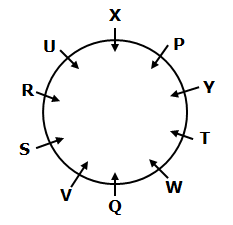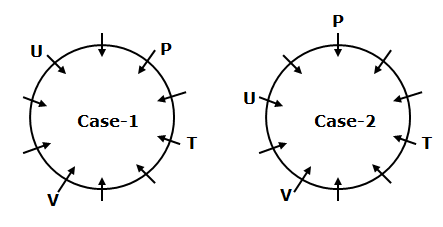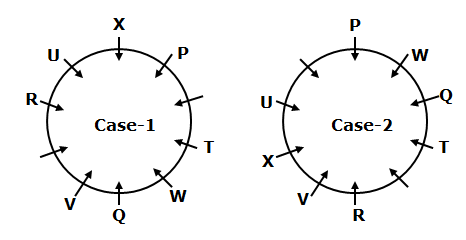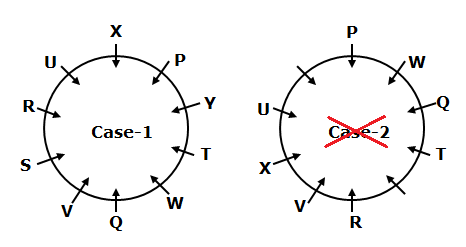# CWC/FCI Prelims 2019 Reasoning Ability Questions (Day-12)

Dear Readers, Exam Race for the Year 2019 has already started, To enrich your preparation here we are providing new series of Practice Questions on Reasoning Ability– Section for CWC/FCI Exam. Aspirants, practice these questions on a regular basis to improve your score in aptitude section. Start your effective preparation from the right beginning to get success in upcoming CWC/FCI Exam.

[WpProQuiz 5523]

Direction (1-5): Read the following information carefully and answer the questions given below.

Ten persons P, Q, R, S, T, U, V, W, X and Y are sitting in a circular table with equal distance from each other. They all are facing centre of the table but not necessary in the same order.

As many persons sitting between X and T is same as W and S. T sits third to the right of V. W sits to the immediate right of Q. P sits second to the left of U and none of them are immediate neighbour of T and V. Three persons are sitting between W and X. R sits second to the right of X.

1) Who among the following sits second to the right of W?

a) S

b) V

c) Y

d) T

e) Q

2) How many persons are sitting between S and P, when counted from left of S?

a) One

b) Two

c) Three

d) More than three

e) None

3) If R is related to T and Q is related to X in a certain way. Then, Y is related to which of the following?

a) W

b) Q

c) V

d) S

e) P

4) Which of the following statements is true?

a) S and Q are immediate neighbours

b) T sits second to the left of P

c) Only two persons are sitting between R and T

d) Q sits opposite to P

e) None is true

5) Four of the following five are alike in a certain way and hence form a group. Which one of the following that does not belong to the group?

a) UV

b) XY

c) SW

d) TX

e) PR

Direction (6-9): These questions are based on five three-digit numbers given below.

264  845  478  729  346

6) If all the even digits are added with ‘1’ and all the odd digits are subtracted by ‘2’ and then arrange the numbers in ascending order from right to left. Then which of the following number will be second from the left end?

a) 729

b) 264

c) 845

d) 346

e) 478

7) What is the resultant of first digit of the largest number is divided by second digit of the second lowest number?

a) 4

b) 1

c) 2

d) 3

e) None of these

8) If in all the odd numbers ‘1’ is added with the tenth digit and in all the even number ‘2’ is subtracted with all the hundredth digit of the given numbers. Then, which of the following will be largest when all the digits within the numbers are added?

a) 845

b) 729

c) 478

d) 346

e) 264

9) If the first and the last digits are interchanged, then what will be the difference between the second lowest and second largest numbers thus formed?

a) 379

b) 412

c) 231

d) 326

e) None of these

10) How many such pair of digits are there in the numbers ‘46817935’ each of which has as many digits between them in the numbers as when they are arranged in descending order?

a) One

b) Two

c) Three

d) Four

e) None of these

Direction (1-5):• T sits third to the right of V. P sits second to the left of U and none of them are immediate neighbour of T and V.• W sits to the immediate right of Q. Three persons are sitting between W and X. R sits second to the right of X.• As many persons sitting between X and T is same as W and S.
• So, Case-2 will be dropped.Direction (6-9):

264  845  478  729  346

375  953  559  537  157

953  559  537  375  157

264  845  478  729  346

8/4=2.

264  845  478  729  346

064  855  278  739  146

264  845  478  729  346

462  548  874  927  643

548~874= 326